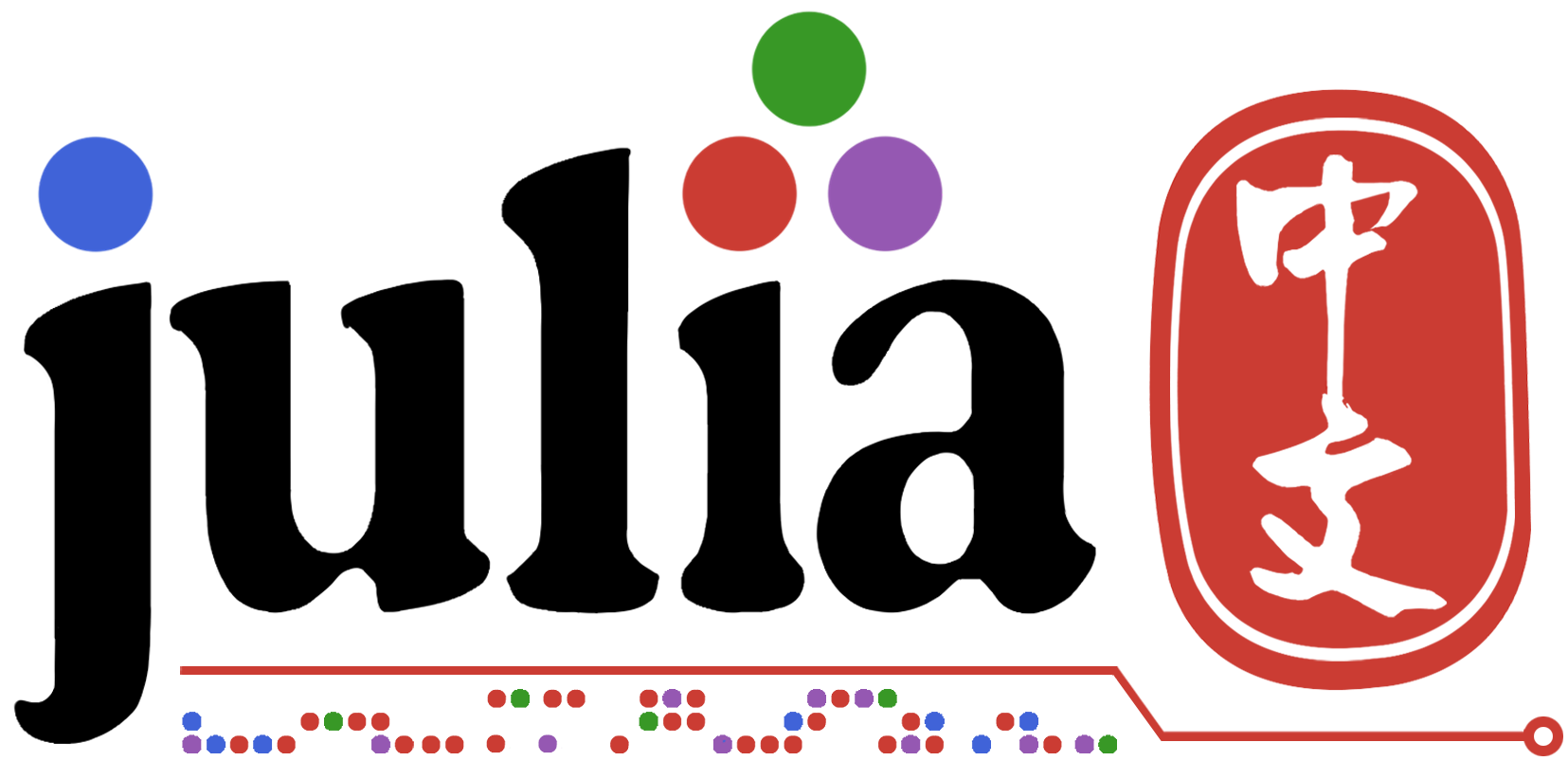# LsqFit拟合

Julia小白，求问一个问题，如何利用LsqFit拟合曲线时使用自定义函数？

``````using LsqFit
function LinearFunc(XData::Vector, Paras::Vector) # A linear function
A, B, = Paras
return XData .* A .+ B
end

# Assume u already have XData and YData
Paras0 = [1.0, 0.0] # Initial Guess
Fit = curve_fit(LinearFunc, XData, YData, Paras0) # Lsq fit
FitYData = LinearFunc(XData, Fit.param) # Generate new Y data to plot
``````

`LinearFunc`的部分可以自行修改，当然也可以修改待定参数的数量。

1 个赞